# Diode Bridge Rectifier

Most of the apparatus we use are AC devices. They require AC power for their operation. We do not have much devices which works on DC supplies. But with the advancement of electronics the DC is gaining its importance as these this devices give an efficient method for AC to DC conversion. Earlier, we used synchronous converter but the conversion process is quite lossy and inefficient. But now electronic devices such as diodes are used for conversion AC to DC. We require DC supply for DC devices Such as computer, battery charger, Etc. All this is possible with the advancement of semiconductor technology.

Now rectifier is a process of conversion to DC to AC. It is the second stage of conversion process. It converts the AC voltage waveform to a rectified voltage.
Now, we have other types of rectification methods that can also be used. So, we may think why should we need this method if other methods are available? The answer to this question is that it provides certain advantages such as no center tap transformer need, high transformer utilization factor, so for the advantages it provides compared to other methods, it is used.

## Principle of Diode Bridge Rectifier

Here we have four diodes connected as shown in the fig. A transformer is used in order to step down the voltage to a desired level at the output a load is connected which consumes power fig:- Bridge rectifier circuit and waveform (rectified). When the upper end of the secondary of transformer is positive, the diode D1 and D3 are forward biased and current flows through them. Current enters through D1 and leaves D3 to the other terminal similarly, during another half cycle, D2 and D4 are forward biased, current enters through D2 and leaves through D4 to the source.

Here, Capacitor is used as a filter which filters out ripple frequencies and provides a DC voltage with less ripple frequency. To get a regulated DC voltage at the output we have to use a voltage regulator after filtering operation.## Mathematical Analysis of Diode Bridge Rectifier

Peak current through the load if diode has forward resistance of RF then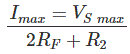Here we get twice the forward resistance. Assuming all the diodes have same forward resistance, two diodes are used for one half cycle twice the forward resistance will come in the expression.

Output currentWhere,
Idc is the DC value of current flowing through the load and Im is the peak value of AC current.
DC output voltage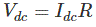Where,
Vdc is the output DC voltage, Idc is the DC current flowing in the circuit and R is the load resistance connected in the circuit.
RMS output current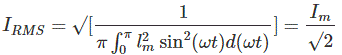RMS output voltage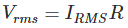Form factor and crest factor
Form factor,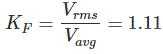Where, Vavg is the average or the DC voltage.
Output FrequencyWhere, fout is the output frequency and fin is the input or supply frequency.
Rectification Efficiency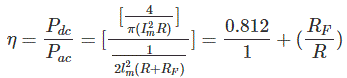Ripple Factor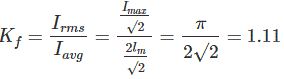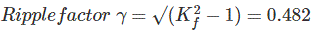TUF Or Transformer Utilization Factor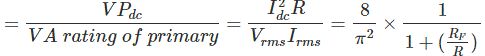## Advantages of Diode Bridge Rectifier

1. Double rectification efficiency then half wave rectifier.
2. Low ripple voltage and higher frequency, so simple filter circuits required.
3. Higher TUF then centre tapped rectifier.
4. No centre tap transformer is required.
5. PIV or Peak Inverse Voltage is one half that of centre tap.

## Disadvantages of Diode Bridge Rectifier

Four diodes required hence the cost of the rectifier will be more.

Want To Learn Faster? 🎓
Get electrical articles delivered to your inbox every week.
No credit card required—it’s 100% free.